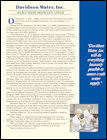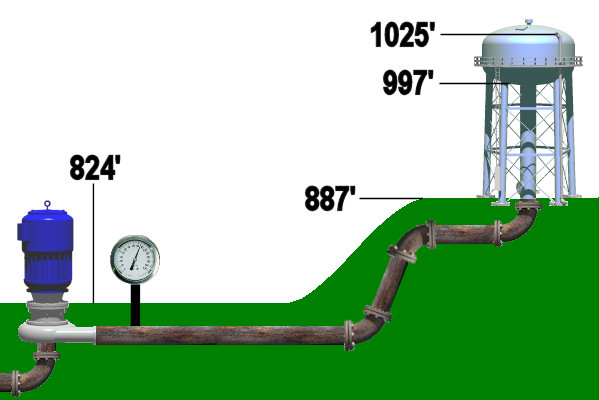Davidson Water 2021 NewsletterArchived Newsletters
 What Determines My Water Pressure? Many customers do not fully understand what controls their water pressure. Several misconceptions regarding this are common, and this typically leads to confusion on the part of the customer.Although there are a few factors that can control pressure, it is primarily the result in the elevation difference between two points. To understand, let’s examine the illustration above. In this illustration, we have a water tank and a pump connected by a water line. The ground elevation at the pump is 824’ above sea level, while the ground elevation at the tank is 887’. The water is stored in the tank in what’s referred to as the “bowl”, which is at the top of the tank. The water level can vary in the tank, but will be in an elevation range (above sea level) between 1025’ and 997’. First, let’s assume the pump at the pump station is off, as we’ll explain it what happens when a pump runs in a moment. If the tank were full, the difference in the elevation between 1025’ (full tank) and 824’ (pump station elevation) would be 201’. Every 2.31 feet of elevation equates to 1 psi of pressure, so dividing 201 by 2.31 gives us a pressure of 87 psi at the pump station. If the bowl was half full (1011’), the result would be 81 psi, while the bottom range of the bowl elevation (997’) would give us a pressure of 75 psi. So the range of pressure at the pump station would normally fall between 87-75 psi. Keep in mind that in peak periods of demand, the customer demand can also pull the pressure down a few psi as well. As demand increases, pressure can be affected. So far, it sounds simple, right? However there is a circumstance where the pressure could be higher than 87 psi. That situation involves a pump running. The pump has the job of refilling the tank with water. In doing so, the pump must boost the pressure higher than the pressure exerted by the tank’s water. This will push water into the tank. In doing this, the pump can provide a pressure higher than the maximum pressure seen by a full tank. In our case, this was 87 psi. So in our case the pump may actually boost the pressure to 95 psi, or higher, while it is running. Once the tank is full, and the pump stops, pressure would once again be dictated by the water level in the tank. While this is a very simplified example, and our system is composed of many tanks, pumps, and nearly 2,000 miles of water line, it should illustrate that pressure is controlled by the ground elevation and the general operation of the system itself.